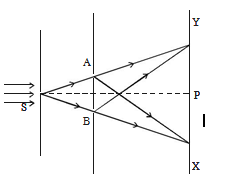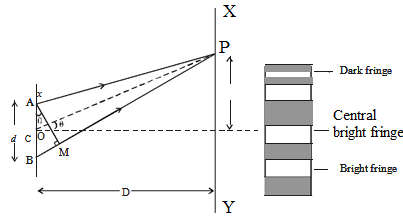# With the help of a diagram, describe the set up of Young’s double slit experiment.Write conditions for the formation of bright and dark fringes in terms of (i) pathdifference (ii) phase difference?

4 years ago

## Double Slit Experiment

The phenomenon of interference was first observed and demonstrated by Thomas Young in 1801. The experimental set up is shown in figure.

Light from a narrow slit S, illuminated by a monochromatic source, is allowed to fall on two narrow slits A and B placed very close to each other. The width of each slit is about 0.03 mm and they are about 0.3 mm apart. Since A and B are equidistant from S, light waves from S reach A and B in phase. So A and B acts as coherent sources.According to Huygen’s principle, wavelets from A and B spread out and overlapping takes place to the right side of AB. When a screen XY is placed at a distance of about 1 meter from the slits, equally spaced alternate bright and dark fringes appear on the screen. These are called interference fringes or bands. Using an eyepiece the fringes can be seen directly. At P on the screen, waves from A and B travel equal distances and arrive in phase. These two waves constructively interfere and bright fringe is observed at P. This is called central bright fringe.

When one of the slits is covered, the fringes disappear and there is uniform illumination on the screen. This shows clearly that the bands are due to interference.

Let d be the distance between two coherent sources A and B of wavelength λ. A screen XY is placed parallel to AB at a distance D from the coherent sources. C is the midpoint of AB. O is a point on the screen equidistant from A and B. P is a point at a distance x from O, as shown in Fig . Waves from A and B meet at P in phase or out of phase depending upon the path difference between two waves.

Draw AM perpendicular to BP

The path difference δ = BP – AP

AP = MP

δ = BP – AP = BP – MP = BMIn right angled ? ABM, BM = d sin θ If θ is small,

sin θ = θ

The path difference δ = θ.d

In right angled triangle COP, tan θ = OP/CO = x/D

For small values of θ, tan θ = θ

Thus, the path difference δ = xd/D

### Bright Fringes

By the principle of interference, condition for constructive interference is the path difference = nλ

xd/D = nλ

Here, n = 0,1,2.....indicate the order of bright fringes

So, x = (D/d) nλ

This equation gives the distance of the nth bright fringe from the point O.

### Dark Fringes

By the principle of interference, condition for destructive interference is the path difference = (2n-1)λ/2

Here, n = 1,2,3 … indicate the order of the dark fringes.

So, x = (D/d) [(2n – 1)λ/2]

This equation gives the distance of the nth dark fringe from the point O. Thus, on the screen alternate dark and bright bands are seen on either side of the central bright band.

### Band Width (β)

The distance between any two consecutive bright or dark bands is called bandwidth.

The distance between (n+1)th and nth order consecutive bright fringes from O is given by,

xn+1 – xn =  [(D/d) [(n+1)λ] –  (D/d) [(n)λ]] = (D/d) λ

Bandwidth, β = (D/d) λ

Similarly, it can be proved that the distance between two consecutive dark bands is also equal to (D/d) λ. Since bright and dark fringes are of same width, they are equi−spaced on either side of central maximum.